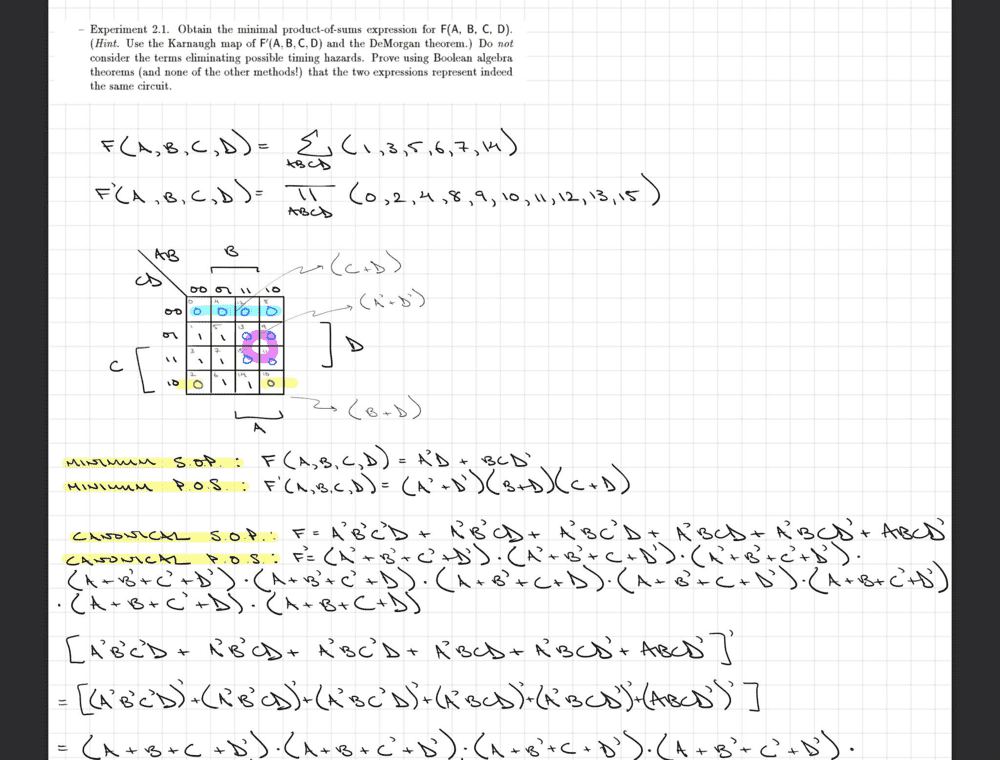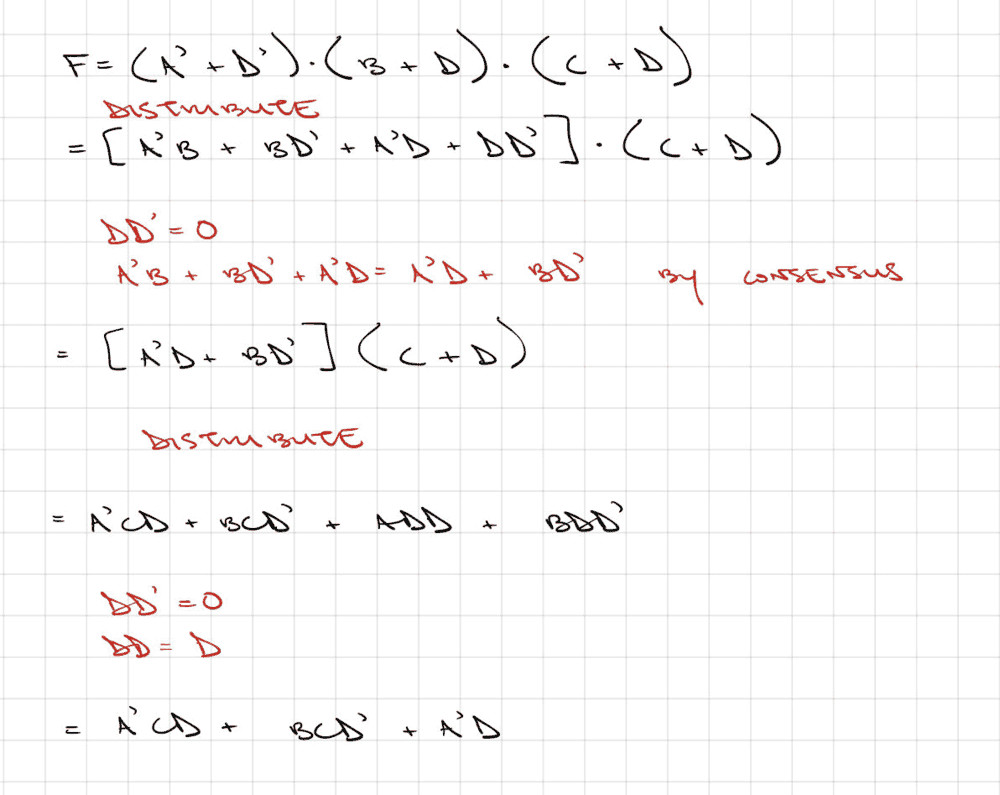# Prove using Boolean algebra that two expressions are equivalent

• Engineering
Homework Statement:
Obtain minimal product of sums expression for F. Using Boolean Algebra, show that the product of sums expression is equivalent to the sum of products expression. Ignore timing hazards.
Relevant Equations:
Boolean algebra and DeMorgan's Laws.Can anyone tell me if I'm doing this right so far? My question really is... does this mean that the next step for me is to expand the last statement shown?Asking because that seems like a lot of work.

andrewkirk
Homework Helper
Gold Member
The way you are going is unnecessarily long, and I don't know whether it can lead to an answer. Forget the canonical forms, which the problem does not ask you to use. Instead focus on the two minimal forms, and try and convert one into the other, using only the Boolean axioms. For me this took nine short lines, starting with the SOP.

Also, note that the LHS of the above statement of the minimal POS contains an error. There should be no prime over the F. The LHS is F(A,B,C,D), the same as for the minimal SOP.

The way you are going is unnecessarily long, and I don't know whether it can lead to an answer. Forget the canonical forms, which the problem does not ask you to use. Instead focus on the two minimal forms, and try and convert one into the other, using only the Boolean axioms. For me this took nine short lines, starting with the SOP.

Also, note that the LHS of the above statement of the minimal POS contains an error. There should be no prime over the F. The LHS is F(A,B,C,D), the same as for the minimal SOP.
I've tried again following your suggestions and I came close to an answer but it's not quite the same expression... there's an extra term. I'm wondering if it may be that my original minimal expressions are incorrect? Could you please take a look at my work below?Is there maybe some kind of 4 variable consensus going on for my last line?

andrewkirk
Homework Helper
Gold Member
Consider the first and last terms.
Can you simplify A'CD + A'D using the Boolean axioms?

Consider the first and last terms.
Can you simplify A'CD + A'D using the Boolean axioms?
Does this simplify to: A'D * [ C + 1 ] = A'D? Since C+1 = 1. If so, I have the expression now and it looks like I've shown I can go from minimal P.O.S. to minimal S.O.P.

Is it also true I can go the other way (i.e. minimal SOP to minimal POS)? I originally tried doing this but it seemed to be more difficult.

Also, you said in the original post that you're not sure if it's possible to convert between the canonical forms... why would that be so? For me, it seems like that should be possible.

Thanks.

andrewkirk
•